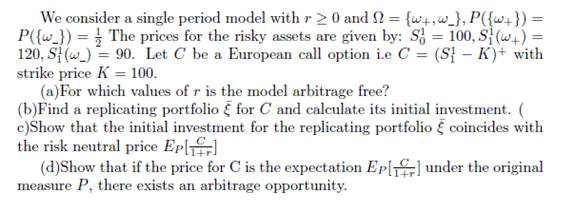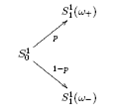Question:(a) Let r be the single period interest rate. The models consists of set Ω = { ω+, ω-} with probability measure,
P({ω+}) = p = ½, P({ω-}) = 1 – p = ½
The stock price can attain two values S11(ω+) = 120 and S11(ω-) = 90 at t = 1For model to be arbitrage free,
S10 = EP [S11] / (1 + r) (1)

Where, EP [S11] = p * S11(ω+) + (1 – p) * S11(ω-)
= ½ * 120 + ½ * 90
= 105 (2)

Therefore, S10 = 100 = 105 / (1 + r)
Þ r = 5%

(b) Let C be a European Call option, and πC is the arbitrage-free price of C at t = 0, then we can define an asset S2 by
S20 = πC and S21 = C
Let us consider a portfolio ξ, where
ξ = (ξ1, ξ2) and S0 = (S10, S20) and V(ω) = ξ . S0 (3)

Here, S21(ω+) = C(ω+) = (S11(ω+) – K)+ = 120 – 100 = 20
S21(ω-) = C(ω-) = (S11(ω-) – K)+ = (90 – 100)+ = 0 (4)

Since the portfolio is risk free, V1(ω+) = V1(ω-) (5)
Þ 120 ξ1 + 20 ξ2 = 90 ξ1 (Using (3))
Þ ξ1 = - ⅔ ξ2

Keeping ξ2 = -1, we get ξ1 = ⅔

Hence, the portfolio ξ = (⅔ , -1) (6)

To calculate the initial investment, we have, V0 = ξ1 S10 + ξ2 S20 (7)
And for risk free portfolio, V1 = V0 (1 + r) (8)

Here, V1 = p V1(ω+) + (1-p) V1(ω-) = V1(ω+) (From (5))

Hence, (⅔ x 100 - πC) (1 + r) = ⅔ x 120 – 20 (Using (4), (6), (7) and (8))
Þ (⅔ x 100 - πC) = 60 / 1.05 = 57.1428
Þ πC = 9.5238

(c) The expected value of call option C at t = 1 is given by
EP [C] = EP [S21] = p * S21(ω+) + (1 – p) * S21(ω-)
Þ EP [C] = EP [S21] = 10 (Using (4)) (9)

Hence, EP [C / (1 + r)] = 10/(1 + r) = 10/1.05 = 9.5238 = πC
Hence prooved.

(d) When C = EP [C / (1 + r)] = 9.5238 under the original measure P, the option is under-priced and arbitrage opportunities exist.

One can borrow an amount equal to 9.5238/ (1 + r) = 9.07 at t = 0 (so that he pays back 9.5238 at t = 1) and buy such an option.

The value of the Option at t = 1 is C = 10, leading to a profit of 10 – 9.5238 = 0.4762 at t=1